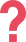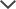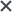## About the NWEA MAP Math Section

The Northwest Evaluation Association (NWEA) is a non-profit organization dedicated to optimizing the quality of education for every student. The Measures of Academic Process (MAP) is its trademark academic aptitude test, designed to adapt to every student’s level, regardless of age. Every grade, ranging from kindergarten to 12th, is required to complete a math section during the test.

While the NWEA math test contains an average of 52 questions, the number can change, as the test adapts itself to the test-taker's abilities. Each section of the math test covers a specific list of topics. The topic choice is based on the student’s grade level, as the test aligns itself with the Common Core. In general, the test covers the following areas based on grade level:

• Number sense
• Estimation and computation
• Algebra
• Geometry
• Measurement
• Statistics and probability
• Problem-solving, reasoning, and proofs

For the higher grades’ MAP math tests, students may need a calculator to help solve some of the problems. In this case, an on-screen calculator will appear. Scratch paper is provided for all grades.

## NWEA MAP Math Sample Questions

All NWEA math test questions are multiple-choice. In some questions, students are asked to fill in missing parts of an equation, while in others, such as word problems, they are given all the information and simply need to work out the equation in their heads or on paper.

For a better understanding of the format in which the material is presented, below are a couple of sample questions:

 Question 1: MAP 4th Grade The following question tests a student's problem-solving skills. It corresponds to an RIT score of 201-210, which is an average fourth-grade score. A snail takes one and a half days to travel one mile.How long would it take a snail to travel two miles? A) 36 hours B) 72 hours C) 3 days D) 4 days E) 4.5 days Answer & Explanation ▼ | ▲ The correct answers are (B) 72 hours and (C) 3 days. You already have the time taken for one mile, so the snail would take double the length of time for two miles. There are 24 hours in a day so in 3 days, you need to multiply 24 by 3. Alternatively, you could add three 24s. 24 × 3 = 72 hours. 24 + 24 + 24 = 72 hours. Therefore, the correct answers are 3 days and 72 hours.
 Question 2: MAP 3rd Grade The following question is a MAP Math geometry sample. This question tests both terminology and deduction skills and corresponds to an RIT score of 191-200, which is an average third-grade score. Select all the triangles that are equilateral. A.B.C.D.E.F.Answer & Explanation ▼ | ▲ The correct answers are (A) and (F). An equilateral triangle has three equal sides and three equal angles. Every angle is 60 degrees. You only need to recognize one of these properties to know it is equilateral. Triangle (A) has two equal angles of 60 degrees. Therefore, to calculate the third angle, subtract the sum of the other two angles from 180 degrees. 60 + 60 = 120. 180 – 120 = 60 degrees. Therefore, the third angle is also 60 degrees, and thus the triangle is equilateral. Triangle (F) has three equal sides, and therefore it is equilateral. The other four triangles do not contain either of these properties and therefore are not equilateral. Triangle (B) has a right-angle and is, therefore, a right triangle. Triangle (C) has two equal sides only and is, therefore, an isosceles triangle. Triangle (D) has no equal sides and is, therefore, a scalene triangle. Triangle (E) has a right-angle symbol and is, therefore, a right triangle.
 Question 3: MAP 6th Grade 6 × 25p = 450What is the value of p? A) 2 B) 3 C) 8 D) 20 E) 75 Answer & Explanation ▼ | ▲ The correct answer is (B). There are a few ways to approach this multiplication equation. One method is to first simplify the left-hand side by multiplying six by 25p. As 6 x 25 = 150, then 6 x 25p = 150p. If you are not confident with the multiplication table for 25, then decompose 25 into 20 and 5. Then, multiply each part by six and then add. 6 x 20 = 120 6 x 5 = 30 Total = 150 Now, rewrite the equation: 150p = 450 // divide both sides by 10* 15p = 45 // divide both sides by three* 5p = 15 // divide both sides by five* p = 3 *If you are able, divide the equation by 150 immediately. If not, divide in batches, as above. Therefore, the correct answer is (B). An alternative approach is to first divide both sides of the equation by six, or by 25, and then proceed.
 Question 4: MAP 6th Grade 6,300 grams =  ? kilograms What is "?" equal to A) 630 B) 63 C) 6.3 D) 63,000 Answer & Explanation ▼ | ▲ The correct answer is (C). There are 1,000 grams in a kilogram. To convert from grams to kilograms, you must divide by 1,000. 6,300 ÷ 1,000 = 6.3 Dividing by 1,000 is the same as dividing by 10 three times: 6,300 ÷ 10 = 630 630 ÷ 10 = 63 63 ÷ 10 = 6.3 In the last division, you need to put in a decimal point as 63 does not end in zero so dividing by 10 makes it a decimal number. Therefore, the correct answer is (C).
 Question 5: MAP 6th Grade Look at the triangle below.What could be a possible measurement for the missing side? Note: Drawing is not to scale   A) 14 B) 13 C) 4 D) 2 Answer & Explanation ▼ | ▲ The correct answer is (C). To solve this question, you need to remember something called the triangular inequality. The triangular inequality states that in a triangle, any side of the triangle must be smaller than the sum of the other sides, and bigger than their difference. Denote the missing length by x. Then, by the triangle inequality, you know that: "8 – 5 = 3 < x < 13 = 8 + 5" So, the missing length is between three and 13. Only one answer choice fulfills the criteria.
 Question 6: MAP 8th Grade Look at the triangle below.What could be a possible measurement for the missing side? Note: Drawing is not to scale   A) \$14 B) \$50 C) \$54 D) \$56 E) \$62 Answer & Explanation ▼ | ▲ The correct answer is (D). There are two ways to solve this question. You can find the discount of each item separately and then add the price after the discount, or you can add the prices of the items together and then calculate the discount from the total price. Examine the second option and add the prices of both items and afterward, calculate the discount. To find the value of a certain percent of a number, multiply this percent (20% in this case) by that number, then divide the result you get by 100. In this case, the two items cost 40 and 30 dollars, meaning that the total price is 70 dollars: (70×20) ÷ 100 = (70 × 10 × 2) ÷ 100 = (700 × 2) ÷ 100 = 1400 ÷ 100 = 14 Now that you know the value of the discount percent (\$14) of the total price (\$70), reduce it from the original price tag and get the current cost during the sale: 70 - 14 = 56 (\$). →Therefore, (D) is the correct answer. Notice that answer (A) is \$14\$, which is the value of the discount, not the total cost of the items after the discount, which is what the question is asking. Alternatively, you can reduce the percentage of discount (20%) from 100% and find the value of the discounted percent of the price: 100% - 20% = 80% and then 80 × 70 ÷ 100 = 56. As mentioned, you can separate the two items (shirt and jeans) and calculate each percent separately and then sum them after the discount. This will be one extra step since there will be two percent calculations to perform instead of only one, but on the other hand, each of them could be simpler.

## NWEA MAP Math Tips

It is quite normal for your child to feel a little anxious about the MAP math section. Math anxiety affects approximately a quarter of the population and starts appearing as early as first grade. However, with the correct amount of practice and motivation, your child can overcome any mathematical doubts he or she may have. Below are some tips you and your child may find useful:

• Practice with sample tests! No matter what, there is no better way to feel more comfortable with a challenge than to expose yourself to it; our MAP math practice tests are designed to gradually make your child feel more and more comfortable with the test material.
• Set a strict studying routine for your child. Making sure your child sticks to a routine ensures better retention of any new information. Large gaps in between study sessions can disrupt your child’s process of gaining new information. Testprep-Online's collection of MAP practice packs comes with math practice quizzes that vary in level, which gives your child the opportunity to progress through an outlined structure.
• Make sure your child eats a healthy meal before the test. On average, the NWEA math test takes about 40 minutes to complete. However, this may vary depending on the student. It is, therefore, important that your child does not arrive on test day with an empty stomach.

## MAP Math Practice

MAP’s math section differs from many other types of math tests, in that there is no ‘risk’. To put it simply, it is impossible to fail an NWEA math test. So why should your child study? Well, with a MAP math score comes a reflection of your child’s academic aptitude in mathematics.

Teachers use a student’s MAP scores to get a holistic understanding of his or her areas of progress. A strong, or above-average score can mean an opportunity for your child to stand out and can ensure further academic growth in the future. In addition, the MAP test is formatted in such a way that one question’s difficulty is dependent on whether the question prior to that one was answered correctly. A student who is unfamiliar with the phrasing of the test, or even something as simple as raw terminology, may get an incorrect answer, and be pushed to a level that, in reality, is far too easy.

Properly preparing for the test, and knowing what to expect, can reduce the risk of misunderstanding a child’s academic ability. Our NWEA MAP practice packs come with three quizzes varying in difficulty, a full-length practice math test, and math enrichment problems to further challenge your child. Within each pack, there are over 200 questions aimed solely at improving your child’s mathematical ability.

Not Sure About Our Packs Yet?

The OLSAT, NNAT, CogAT, New York City Gifted and Talented Test, MAP, and other trademarks are the property of their respective trademark holders. None of the trademark holders are affiliated with TestPrep-Online or this website.

Need HelpNeed Help